# Calculus 3 : Normal Vectors

## Example Questions

### Example Question #31 : Normal Vectors

Determine whether the two vectors,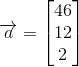and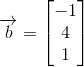, are orthogonal or not.

The two vectors are orthogonal.

The two vectors are not orthogonal.

The two vectors are not orthogonal.

Explanation:

Vectors can be said to be orthogonal, that is to say perpendicular or normal, if their dot product amounts to zero: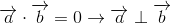To find the dot product of two vectors given the notation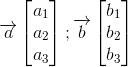Simply multiply terms across rows: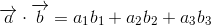For our vectors,and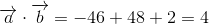The two vectors are not orthogonal.

### Example Question #32 : Normal Vectors

Determine whether the two vectors,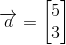and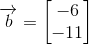, are orthogonal or not.

The two vectors are orthogonal.

The two vectors are not orthogonal.

The two vectors are not orthogonal.

Explanation:

Vectors can be said to be orthogonal, that is to say perpendicular or normal, if their dot product amounts to zero:To find the dot product of two vectors given the notation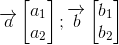Simply multiply terms across rows: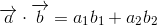For our vectors,and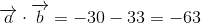The two vectors are not orthogonal.

### Example Question #33 : Normal Vectors

Determine whether the two vectors,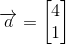and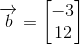, are orthogonal or not.

The two vectors are orthogonal.

The two vectors are not orthogonal.

The two vectors are orthogonal.

Explanation:

Vectors can be said to be orthogonal, that is to say perpendicular or normal, if their dot product amounts to zero:To find the dot product of two vectors given the notationSimply multiply terms across rows:For our vectors,and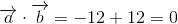The two vectors are orthogonal.

### Example Question #34 : Normal Vectors

Determine whether the two vectors,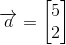and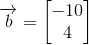, are orthogonal or not.

The two vectors are orthogonal.

The two vectors are not orthogonal.

The two vectors are not orthogonal.

Explanation:

Vectors can be said to be orthogonal, that is to say perpendicular or normal, if their dot product amounts to zero:To find the dot product of two vectors given the notationSimply multiply terms across rows:For our vectors,and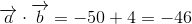The two vectors are not orthogonal.

### Example Question #35 : Normal Vectors

Determine whether the two vectors,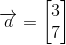and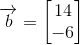, are orthogonal or not.

The two vectors are not orthogonal.

The two vectors are orthogonal.

The two vectors are orthogonal.

Explanation:

Vectors can be said to be orthogonal, that is to say perpendicular or normal, if their dot product amounts to zero:To find the dot product of two vectors given the notationSimply multiply terms across rows:For our vectors,and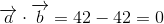The two vectors are orthogonal.

### Example Question #36 : Normal Vectors

Determine whether the two vectors,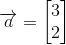and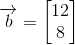, are orthogonal or not.

The two vectors are not orthogonal.

The two vectors are orthogonal.

The two vectors are not orthogonal.

Explanation:

Vectors can be said to be orthogonal, that is to say perpendicular or normal, if their dot product amounts to zero:To find the dot product of two vectors given the notationSimply multiply terms across rows:For our vectors,and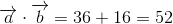The two vectors are not orthogonal.

### Example Question #37 : Normal Vectors

Determine whether the two vectors,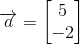and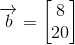, are orthogonal or not.

The two vectors are orthogonal.

The two vectors are not orthogonal.

The two vectors are orthogonal.

Explanation:

Vectors can be said to be orthogonal, that is to say perpendicular or normal, if their dot product amounts to zero:To find the dot product of two vectors given the notationSimply multiply terms across rows:For our vectors,and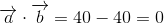The two vectors are orthogonal.

### Example Question #38 : Normal Vectors

Determine whether the two vectors,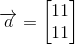and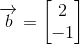, are orthogonal or not.

The two vectors are orthogonal.

The two vectors are not orthogonal.

The two vectors are not orthogonal.

Explanation:

Vectors can be said to be orthogonal, that is to say perpendicular or normal, if their dot product amounts to zero:To find the dot product of two vectors given the notationSimply multiply terms across rows:For our vectors,and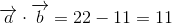The two vectors are not orthogonal.

### Example Question #39 : Normal Vectors

Determine whether the two vectors,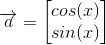and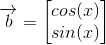, are orthogonal or not.

The two vectors are orthogonal.

It cannot be determined unlessis known.

The two vectors are not orthogonal.

The two vectors are not orthogonal.

Explanation:

Vectors can be said to be orthogonal, that is to say perpendicular or normal, if their dot product amounts to zero:To find the dot product of two vectors given the notationSimply multiply terms across rows:For our vectors,and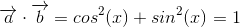The two vectors are not orthogonal.

### Example Question #40 : Normal Vectors

Determine whether the two vectors,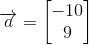and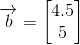, are orthogonal or not.

The two vectors are not orthogonal.

The two vectors are orthogonal.

The two vectors are orthogonal.

Explanation:

Vectors can be said to be orthogonal, that is to say perpendicular or normal, if their dot product amounts to zero:To find the dot product of two vectors given the notationSimply multiply terms across rows:For our vectors,and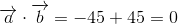The two vectors are orthogonal.